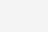# Python numpy有哪些功能优于列表1、与列表相比，numpy具有更自然、更方便的数学运算集成。

```a = [6, 2, 1, 4, 3]
b = [e + 1 for e in a]```

```a = np.array([6, 2, 1, 4, 3])
b = a + 1```

2、numpy也适用于每个numpy数学函数：例如，可以使用列表中每个元素的指数。

```a = [6, 2, 1, 4, 3]
b = [1, 2, 3, 2, 3]
c = [e1 + e2 for (e1, e2) in zip(a, b)]```